The ANOVA Procedure

Computational Method

Letrepresent the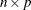design matrix. The columns ofcontain only 0s and 1s. Let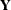represent the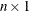vector of dependent variables.

In the GLM procedure,,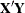, andare formed in main storage. However, in the ANOVA procedure, only the diagonals ofare computed, along withand. Thus, PROC ANOVA saves a considerable amount of storage as well as time. The memory requirements for PROC ANOVA are asymptotically linear functions of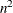and, where n is the number of dependent variables and r the number of independent parameters.

The elements ofare cell totals, and the diagonal elements ofare cell frequencies. Since PROC ANOVA automatically pools omitted effects into the next higher-level effect containing the names of the omitted effect (or within-error), a slight modification to the rules given by Searle (1971, p. 389) is used.

1. PROC ANOVA computes the sum of squares for each effect as if it is a main effect. In other words, for each effect, PROC ANOVA squares each cell total and divides by its cell frequency. The procedure then adds these quantities together and subtracts the correction factor for the mean (total squared over N).

2. For each effect involving two CLASS variable names, PROC ANOVA subtracts the SS for any main effect with a name that is contained in the two-factor effect.

3. For each effect involving three CLASS variable names, PROC ANOVA subtracts the SS for all main effects and two-factor effects with names that are contained in the three-factor effect. If effects involving four or more CLASS variable names are present, the procedure continues this process.